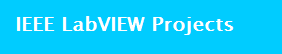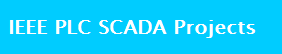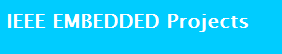Project Description It's relatively simple to use an Arduino to measure voltages. The Arduino has several analog input pins that connect to an analog-to-digital converter (ADC) inside the Arduino. The Arduino ADC is a ten-bit converter, meaning that the output value will range from 0 to 1023. We will obtain this value by using the analogRead() function. If you know the reference voltage--in this case we will use 5 V--you can easily calculate the voltage present at the analog input. To display the measured voltage, we will use a liquid crystal display (LCD) that has two lines of 16 characters. LCDs are widely used to display data by devices such as calculators, microwave ovens, and many other electrical appliances. This project will also show you how to measure voltages above the reference voltage by using a voltage divider.
Technologies Used
arduino microcontroller,power supply,serial comm,volt sensor,lcd
For..
BE and B.Tech Engineering Students, Diploma Students

#### Fill the form to get more details of the project